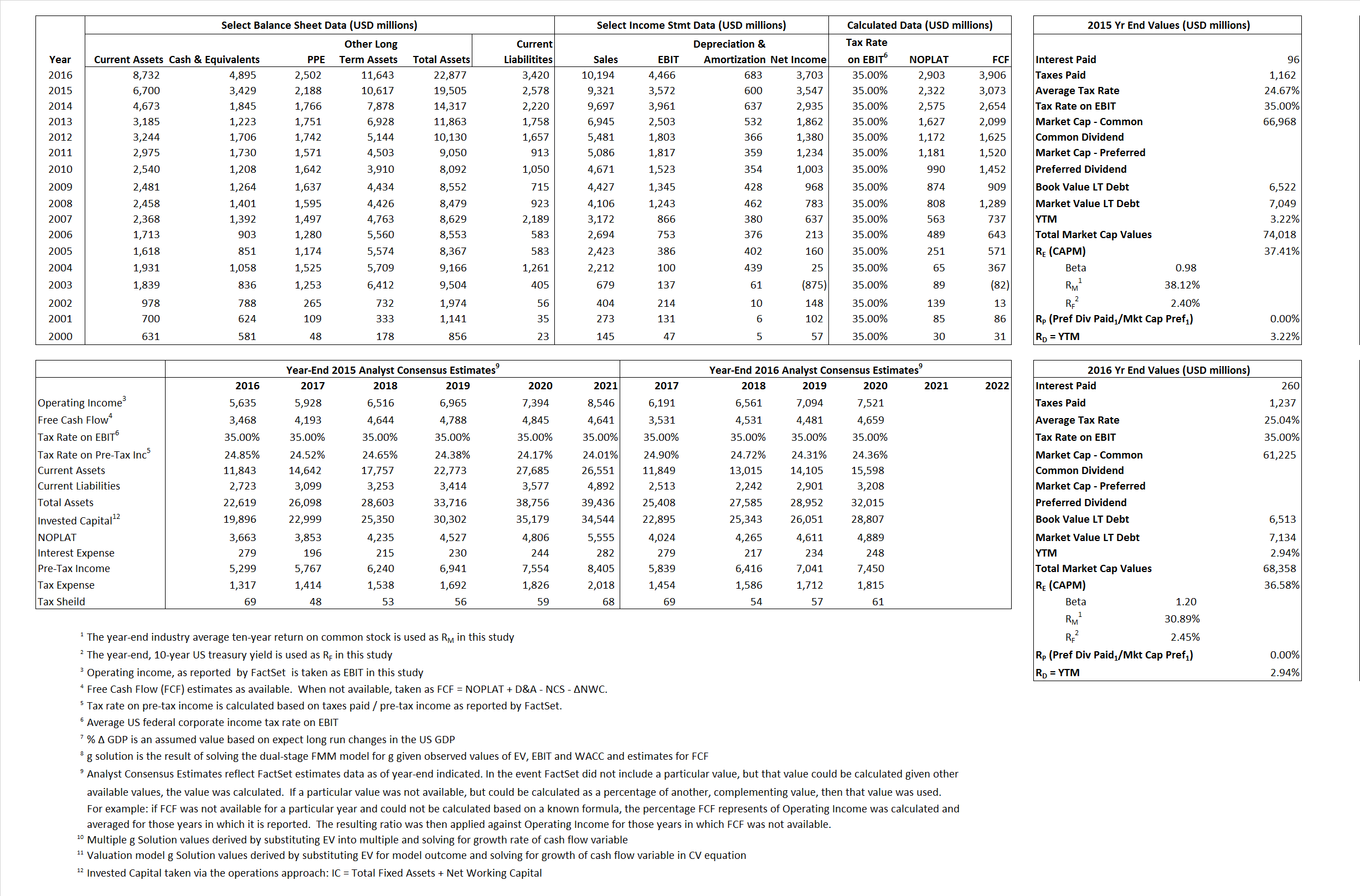# Biogen Idec

## Analyst Listing

The following analysts provide coverage for the subject firm as of May 2016:

 Broker Analyst Analyst Email Credit Suisse Alethia Young alethia.young@credit-suisse.com HC Wainwright Andrew S. Fein afein@hcwresearch.com Jefferies Brian Abrahams babrahams@jefferies.com Raymond James Christopher J. Raymond chris.raymond@raymondjames.com Cowen & Company Eric Schmidt eric.schmidt@cowen.com Leerink Partners Geoffrey C. Porges geoffrey.porges@leerink.com Wells Fargo Securities Jim Birchenough jim.birchenough@wellsfargo.com William Blair John Sonnier jsonnier@williamblair.com Piper Jaffray Joshua Schimmer joshua.e.schimmer@pjc.com BMO Capital Markets M. Ian Somaiya ian.somaiya@bmo.com Evercore ISI Mark Schoenebaum mark.schoenebaum@evercoreisi.com RBC Capital Markets Michael J. Yee michael.yee@rbccm.com Atlantic Equities Steve Chesney s.chesney@atlantic-equities.com Stifel Nicolaus Thomas Shrader shradert@stifel.com Guggenheim Securities William Tanner william.tanner@guggenheimpartners.com

## Primary Input Data## Derived Input Data

### Equational Form

Net Operating Profit Less Adjusted Taxes NOPLAT 2,322  2,903$NOPLAT\, =\, EBIT\, x\, (1 \,-\, Avg \,\,Tax\,\, Rate\,\, on\,\, EBIT)$
Free Cash Flow FCF 3,073 3,906$FCF\,=NOPLAT\,+\,Non-Cash\,Expenses-\Delta NWC\,-\,NCS$
Tax Shield TS 24 65$TS\,=\,Interest\,\,Paid\,\,x\,\, Avg \,\,Tax\,\,Rate\,\, on\,\, Pre-Tax\,\, Income$
Invested Capital IC 16,927 19,457$IC\,=\,Fixed\,\,Operating\,\,Assets\,\,+\,\,Net\,\, Working\,\, Capital$
Return on Invested Capital ROIC 13.72% 14.92%$ROIC\,=\,\frac { NOPLAT }{ IC }$
Net Investment NetInv 5,431 3,213$NetInv\,=\,{ {IC}_{1}}-{{IC}_{0}}+Depreciation$
Investment Rate IR 233.90% 110.68%$IR\,=\,\frac {NetInv}{NOPLAT}$
Weighted Average Cost of Capital
WACCMarket 34.07% 32.99%$WACC\,=\,\frac { E }{ V } { R }_{ E }\,+\,\frac { P }{ V } { R }_{ P }\,+\,\frac { D }{ V } { R }_{ D }\left( 1- Avg\,\, Tax\,\,Rate\,\,on\,\,Pre-Tax\,\,Income \right)$
WACCBook  8.63%  9.54%
Enterprise value
EVMarket 70,589  63,463$EV\,=\,Market\,\,Cap\,\,Equity\,+\,\,Long\,\,Term\,\,Debt\,-\,Cash$
EVBook  70,061  62,843
Long-Run Growth
g = IR x ROIC
32.08%  16.51% Long-run growth rates of the income variable are used in the Continuing Value portion of the valuation models.
g = %$\Delta$ GDP  2.50%  2.50%
Margin from Operations M 38.32%  43.81%$M\,\,=\,\,\frac{EBIT}{SALES}$
Depreciation/Amortization Rate D 14.39%  13.26%$D\,\,=\,\,\frac{D+A}{EBITDA}$

## Valuation Multiple Outcomes

The outcomes presented in this study are the result of original input data, derived data, and synthesized inputs.

### model g solution

12/31/2015 12/31/2016 12/31/2015 12/31/2016 12/31/2015 12/31/2016

EV/SALES$\frac {EV}{Sales} \,= \,\frac{ROIC\, -\, g}{ROIC\,(WACC\,-\,g)}\,(1\,-\,T)\,(M)$

7.57 6.23 40.50% 40.98% 35.46% 34.95%

EV/EBITDA$\frac {EV}{EBITDA} \,= \,\frac{ROIC\, -\, g}{ROIC\,(WACC\,-\,g)}\,(1\,-\,T)\,(1\,-\,D)$

16.92 12.33  40.50% 40.98% 35.46% 34.95%

EV/NOPLAT$\frac {EV}{NOPLAT} \,= \,\frac{ROIC\, -\, g}{ROIC\,(WACC\,-\,g)}$

30.40 21.86  40.50% 40.98% 35.46% 34.95%

EV/FCFOPS$\frac {EV}{FCF_{OPS}} \,= \,\frac{ROIC\, -\, g}{ROIC\,(WACC\,-\,g)}\,(1\,-\,T)$

22.97 16.25  40.50% 40.98% 35.46% 34.95%

EV/EBIT$\frac {EV}{EBIT} \,= \,\frac{ROIC\, -\, g}{ROIC\,(WACC\,-\,g)}\,(1\,-\,T)$

19.76 14.21  40.50% 40.98% 35.46% 34.95%

EV/IC$\frac {EV}{IC} \,= \,\frac{ROIC\, -\, g}{WACC\,-\,g}$

4.17 3.26  40.50% 40.98% 35.46% 34.95%